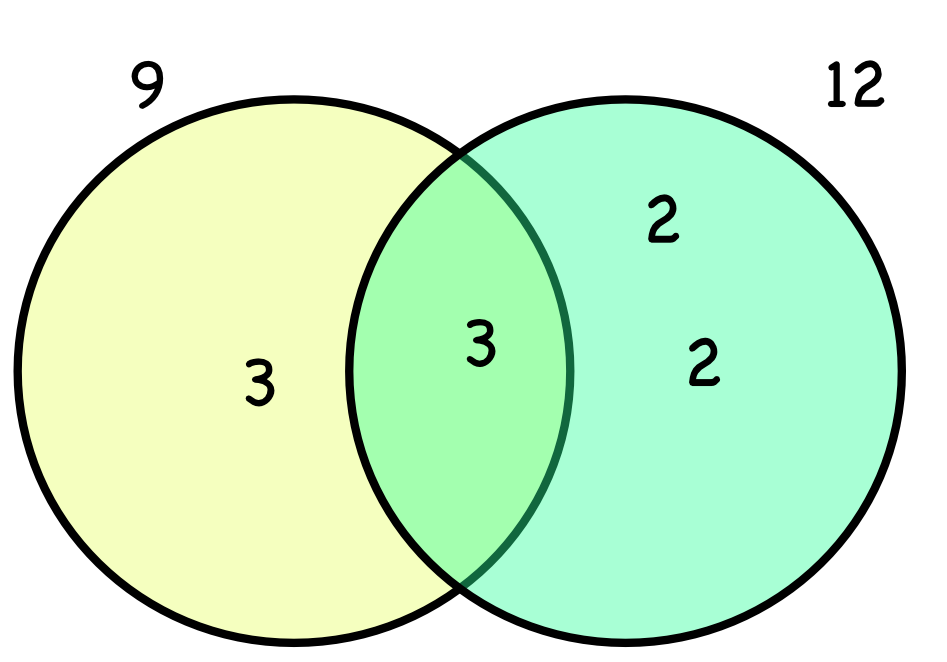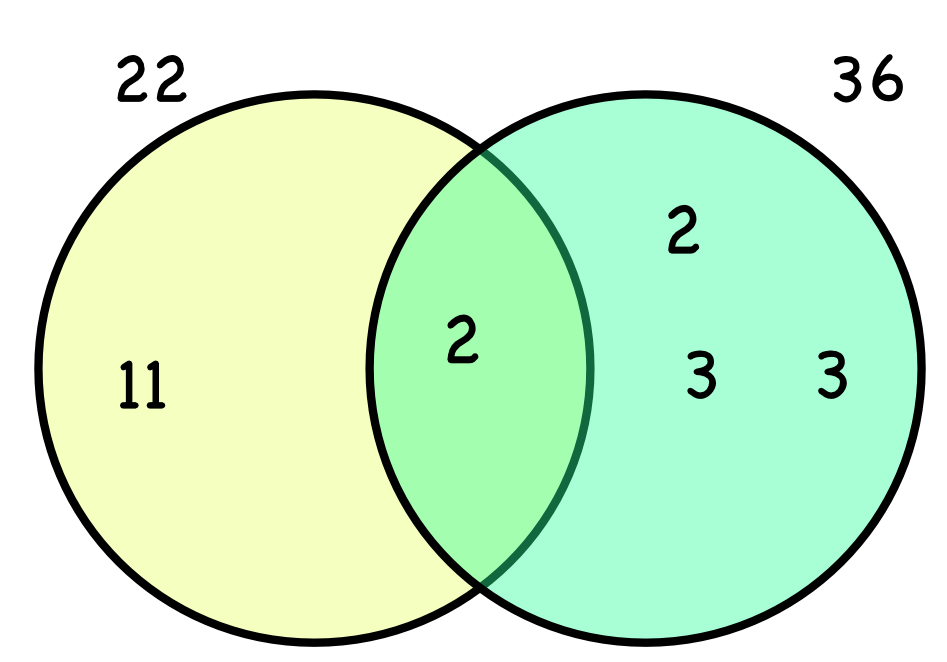Lowest Common Multiple

## Lowest Common Multiple

Multiples can be shared by numbers. The lowest multiple shared by two numbers is called the Lowest Common Multiple, or LCM.

multiples of 9 are: 9, 18, 27, 36, 45, 54, 63, 72, ...

multiples of 12 are: 12, 24, 36, 48, 60, 72, ...

Multiples that appear in both lists are 36 and 72. The Lowest Common Multiple is 36, which is the first multiple that 9 and 12 share.

For larger numbers, it is easier to determine the LCM by using a Venn Diagram. Using a Prime Factor Tree, determine the product of the primes for each number.

Place these prime factors in a Venn Diagram. The union of the two sets represents the lowest common multiple. This can be written as for example) 9 ∪ 12, or 9 union 12.The LCM (multiply each number on the Venn Diagram) = 2 x 2 x 3 x 3 = 36.

Higher/Extended only:To find the Lowest Common Multiple of more than two numbers, create a Venn Diagram with a set of prime factors for each number. The union of ALL the sets will give the factors for the Lowest Common Multiple for ALL the numbers.

## Example 1

What is the LCM of 8 and 10?

By inspection:

Factors of 8: 8, 16, 24, 32, 40, 48, 56, 64, 72, 80

Factors of 10: 10, 20, 30, 40, 50, 60

The lowest common multiple is 40.

## Example 2

In a game, one type of alien appears ever 22 seconds. A second type of alien appears every 36 seconds. If they have just appeared together, how long will it be until they appear together again?

This is a Lowest Common Multiple question. Using prime factors:

22: 2 x 11

36: 2 x 2 x 3 x 3

LCM = 2 x 2 x 3 x 3 x 11 = 396.

Check that 22 and 36 both divide into 396 without leaving a remainder.✔